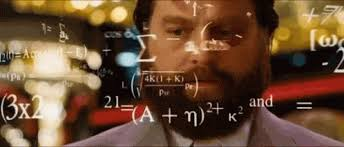# Tips to learn difficult formulas.

Does the maze of alpha, beta, gamma, mix in your head and you end up screwing the entire question? Formula-based questions, require you the judgment to be able to apply the correct formula. Formulas could be a pain, we know. But, with these tips ace the formula maze!2. Practice: That’s the key, practice as much as you can. Do not flip pages of the book, when you need the formula to solve a question. Spend a minute and try recollecting. You will err in the few initial attempts, but then you will just be fine.3. Not what the formula is, but what it says: There is a derivative theorem behind every formula. The formulas are very practically derived. If at the very end moment, it skips your mind, invest some time to practically think what the solution-formula should be.